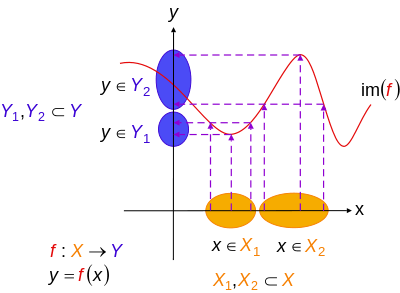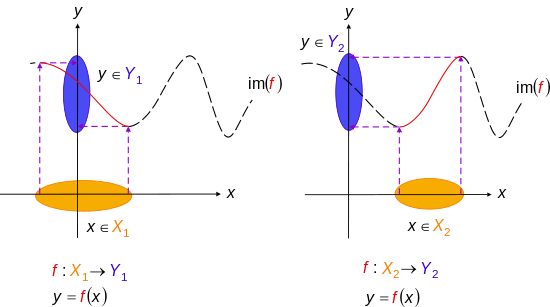# Injective function

"Injective" redirects here. For injective modules, see Injective module.An injective non-surjective function (injection not a bijection)An injective surjective function (bijection)A non-injective surjective function (surjection not a bijection)

In mathematics, an injective function or injection or one-to-one function is a function that preserves distinctness: it never maps distinct elements of its domain to the same element of its codomain. In other words, every element of the function's codomain is the image of at most one element of its domain. The term one-to-one function must not be confused with one-to-one correspondence (aka bijective function), which uniquely maps all elements in both domain and codomain to each other, (see figures).

Occasionally, an injective function from X to Y is denoted f: XY, using an arrow with a barbed tail (U+21A3 RIGHTWARDS ARROW WITH TAIL). The set of injective functions from X to Y may be denoted YX using a notation derived from that used for falling factorial powers, since if X and Y are finite sets with respectively m and n elements, the number of injections from X to Y is nm (see the twelvefold way).

A function f that is not injective is sometimes called many-to-one. However, the injective terminology is also sometimes used to mean "single-valued", i.e., each argument is mapped to at most one value.

A monomorphism is a generalization of an injective function in category theory.

## Definition

For more details on notation, see Function (mathematics) § Notation.

Let f be a function whose domain is a set A. The function f is injective if and only if for all a and b in A, if f(a) = f(b), then a = b; that is, f(a) = f(b) implies a = b.  Equivalently, if ab, then f(a) ≠ f(b).

Symbolically,which is logically equivalent to the contrapositive,## Examples

• For any set X and any subset S of X the inclusion map SX (which sends any element s of S to itself) is injective. In particular the identity function XX is always injective (and in fact bijective).
• If the domain X = or X has only one element, the function XY is always injective.
• The function f : R  R defined by f(x) = 2x + 1 is injective.
• The function g : R  R defined by g(x) = x2 is not injective, because (for example) g(1) = 1 = g(−1). However, if g is redefined so that its domain is the non-negative real numbers [0,+∞), then g is injective.
• The exponential function exp : RR defined by exp(x) = ex is injective (but not surjective as no real value maps to a negative number).
• The natural logarithm function ln : (0, ∞) → R defined by x ln x is injective.
• The function g : R  R defined by g(x) = xn x is not injective, since, for example, g(0) = g(1) = 0.

More generally, when X and Y are both the real line R, then an injective function f : R  R is one whose graph is never intersected by any horizontal line more than once. This principle is referred to as the horizontal line test.Injective functions. Diagramatic interpretation in the Cartesian plane, defined by the mapping f : XY, where y = f(x), X = domain of function, Y = range of function, and im(f) denotes image of f. Every one x in X maps to exactly one unique y in Y. The circled parts of the axes represent domain and range sets – in accordance with the standard diagrams above.Not an injective function. Here X1 and X2 are subsets of X, Y1 and Y2 are subsets of Y: for two regions where the function is not injective because more than one domain element can map to a single range element. That is, it is possible for more than one x in X to map to the same y in Y.Making functions injective. The previous function f : XY can be reduced to one or more injective functions (say) f : X1Y1 and f : X2Y2, shown by solid curves (long-dash parts of initial curve are not mapped to anymore). Notice how the rule f has not changed – only the domain and range. X1 and X2 are subsets of X, Y1 and Y2 are subsets of R: for two regions where the initial function can be made injective so that one domain element can map to a single range element. That is, only one x in X maps to one y in Y.

## Injections can be undone

Functions with left inverses are always injections. That is, given f : X  Y, if there is a function g : Y  X such that, for every x X

g(f(x)) = x (f can be undone by g)

then f is injective. In this case, g is called a retraction of f. Conversely, f is called a section of g.

Conversely, every injection f with non-empty domain has a left inverse g (in conventional mathematics). Note that g may not be a complete inverse of f because the composition in the other order, f o g, may not be the identity on Y. In other words, a function that can be undone or "reversed", such as f, is not necessarily invertible (bijective). Injections are "reversible" but not always invertible.

Although it is impossible to reverse a non-injective (and therefore information-losing) function, one can at least obtain a "quasi-inverse" of it, that is a multiple-valued function.

## Injections may be made invertible

In fact, to turn an injective function f : X  Y into a bijective (hence invertible) function, it suffices to replace its codomain Y by its actual range J = f(X). That is, let g : X  J such that g(x) = f(x) for all x in X; then g is bijective. Indeed, f can be factored as inclJ,Y o g, where inclJ,Y is the inclusion function from J into Y.

More generally, injective partial functions are called partial bijections.

## Other properties

• If f and g are both injective, then f o g is injective.The composition of two injective functions is injective.
• If g o f is injective, then f is injective (but g need not be).
• f : X  Y is injective if and only if, given any functions g, h : W  X, whenever f o g = f o h, then g = h. In other words, injective functions are precisely the monomorphisms in the category Set of sets.
• If f : X  Y is injective and A is a subset of X, then f 1(f(A)) = A. Thus, A can be recovered from its image f(A).
• If f : X  Y is injective and A and B are both subsets of X, then f(A  B) = f(A)  f(B).
• Every function h : W  Y can be decomposed as h = f o g for a suitable injection f and surjection g. This decomposition is unique up to isomorphism, and f may be thought of as the inclusion function of the range h(W) of h as a subset of the codomain Y of h.
• If f : X  Y is an injective function, then Y has at least as many elements as X, in the sense of cardinal numbers. In particular, if, in addition, there is an injection fromto, thenandhave the same cardinal number. (This is known as the Cantor–Bernstein–Schroeder theorem.)
• If both X and Y are finite with the same number of elements, then f : X  Y is injective if and only if f is surjective (in which case f is bijective).
• An injective function which is a homomorphism between two algebraic structures is an embedding.
• Unlike surjectivity, which is a relation between the graph of a function and its codomain, injectivity is a property of the graph of the function alone; that is, whether a function f is injective can be decided by only considering the graph (and not the codomain) of f.

## Proving that functions are injective

A proof that a function ƒ is injective depends on how the function is presented and what properties the function holds. For functions that are given by some formula there is a basic idea. We use the contrapositive of the definition of injectivity, namely that if ƒ(x) = ƒ(y), then x = y.

Here is an example:

ƒ = 2x + 3

Proof: Let ƒ : X  Y. Suppose ƒ(x) = ƒ(y). So 2x + 3 = 2y + 3 => 2x = 2y => x = y. Therefore it follows from the definition that ƒ is injective.

There are multiple other methods of proving that a function is injective. For example, in calculus if ƒ is a differentiable function defined on some interval, then it is sufficient to show that the derivative is always positive or always negative on that interval. In linear algebra, if ƒ is a linear transformation it is sufficient to show that the kernel of ƒ contains only the zero vector. If ƒ is a function with finite domain it is sufficient to look through the list of images of each domain element and check that no image occurs twice on the list.

## Notes

1. "Unicode" (PDF). Retrieved 2013-05-11.
2. This principle is valid in conventional mathematics, but may fail in constructive mathematics. For instance, a left inverse of the inclusion {0,1} → R of the two-element set in the reals violates indecomposability by giving a retraction of the real line to the set {0,1}.
3. Williams, Peter. "Proving Functions One-to-One".

## ReferencesWikimedia Commons has media related to Injectivity.Look up injective in Wiktionary, the free dictionary.